W.J. Riley, Hamilton Technical Services

ABSTRACT

This tutorial paper is an introduction to the Hadamard variance as it is applied for the analysis of frequency stability.  It reviews the classical Hadamard variance, describes an overlapping version of the Hadamard variance that offers better estimates for this statistic, introduces a modified version of the Hadamard variance, and the newer Hadamard total variance that offers improved confidence at large averaging factors. The setting of confidence limits and the recognition of divergent power law noise types is also discussed.

BACKGROUND

The Hadamard  variance is based on the Hadamard transform , which was adapted by Baugh as the basis of a time-domain measure of frequency stability .  As a spectral estimator, the Hadamard transform has higher resolution than the Allan variance , since the equivalent noise bandwidth of the Hadamard and Allan spectral windows are 1.2337N-1t-1 and 0.476t-1 respectively .  For the purposes of time-domain frequency stability characterization, the most important advantage of the Hadamard variance is its insensitivity to linear frequency drift, making it particularly useful for the analysis of rubidium atomic clocks [6, 7].  It has also been used as one of the components of a time-domain multivariance analysis , and is related to the 3rd structure function of phase noise [8, 21].

The Hadamard variance is a 3-sample variance, as commonly used in the frequency control community , with binomially-weighted coefficients that is similar to the 2-sample Allan variance. It examines the 2nd difference of the fractional frequencies, the 3rd difference of the phase variations. Because of this, the Hadamard variance, HVAR or s²H(t), converges for the Flicker Walk FM (a = -3) and Random Run FM (a = -4) power-law noise types. It is also unaffected by linear frequency drift.  For frequency data, the Hadamard variance is defined as: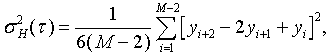where yi is the ith of M fractional frequency values at averaging time t.

For phase data, the Hadamard variance is defined as: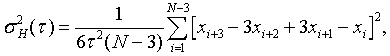where xi is the ith of N=M+1 phase values at averaging time t.

Like the Allan variance, the Hadamard variance is usually expressed as its square-root, the Hadamard deviation, HDEV or sH(t).

In the same way that the overlapping Allan variance makes maximum use of a data set by forming all possible fully overlapping 2-sample pairs at each averaging time t, the overlapping Hadamard variance uses all 3-sample combinations . It can be estimated from a set of M frequency measurements for averaging time t = mt0, where m is the averaging factor and t0 is the basic measurement interval, by the expression: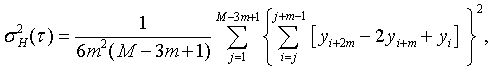where yi is the ith of M fractional frequency values at each measurement time.

In terms of phase data, the overlapping Hadamard variance can be estimated from a set of N = M+1 time measurements as: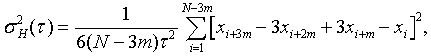where xi is the ith of N=M+1 phase values at each measurement time.

Computation of the overlapping Hadamard variance is more efficient for phase data, where the averaging is accomplished by simply choosing the appropriate interval. For frequency data, an inner averaging loop over m frequency values is necessary.  The result is usually expressed as the square root, Hsy(t), the Hadamard deviation, HDEV. The expected value of the overlapping statistic is the same as the normal one described above, but the confidence interval of the estimation is better. Even though all the additional overlapping differences are not statistically independent, they nevertheless increase the number of degrees of freedom and thus improve the confidence in the estimation. Analytical methods are available for calculating the number of degrees of freedom for an overlapping Allan variance estimation, and that same theory can be used to establish reasonable single or double-sided confidence intervals for an overlapping Hadamard variance estimate with a certain confidence factor, based on Chi-squared statistics.

Sample variances are distributed according to the expression:

c²(p, df) =(df · s²) / s²

where c² is the Chi-square value for probability p and degrees of freedom df, s² is the sample variance, s² is the true variance, and df is the # of degrees of freedom (not necessarily an integer). The df is determined by the number of data points and the noise type. Given the df, the confidence limits around the measured sample variance are given by

s²min = (s2 · df) / c²(p, df), and  s²max = (s2 · df) / c²(1-p, df).

By similarity to the modified Allan variance, a modified version of the Hadamard variance can be defined  which employs averaging of the phase data over the m adjacent samples that define the analysis t= m·t0. In terms of phase data, the 3-sample modified Hadamard variance is defined as: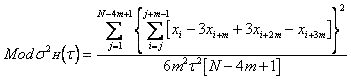where N is the number of phase data points xi at the sampling interval t0, and m is the averaging factor, which can extend from 1 to (floor)N/4 . This is an unbiased estimator of the modified Hadamard variance, MHVAR. Expressions for the equivalent number of c² degrees of freedom (edf) required to set MHVAR confidence limits are available in .

Clock noise (and other noise processes) can be described in terms of power spectral density, which can be modeled as a power law function S(fa), where f is Fourier frequency and a is the power law exponent. When a variance such as MHVAR is plotted on log-log axes versus averaging time, the various power law noises correspond to particular slopes . MHVAR was developed in Reference  for determining the power law noise type of Internet traffic statistics, where it was found to be slightly better for that purpose than the modified Allan variance, MAVAR, when there were a sufficient number of data points. MHVAR could also be useful for frequency stability analysis, perhaps in cases where it was necessary to distinguish between short-term white and flicker PM noise in the presence of more divergent (a = -3 and -4) flicker walk and random run FM noises. The MHVAR log-log slope is related to the power law noise exponent by m = -3 - a.

The modified Hadamard variance concept can be generalized to subsume AVAR, HVAR, MAVAR, MHVAR, and MHVARs using higher-order differences: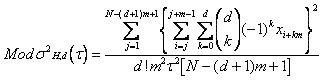where d = phase differencing order; d = 2 corresponds to MAVAR, d = 3 to MHVAR; higher order differencing is not commonly used in the field of frequency stability analysis. The unmodified, non-overlapped AVAR and HVAR variances are given by setting m = 1. The allowable power law exponent for convergence of the variance is equal to a > 1 - 2d, so the 2nd difference Allan variances can be used for a > -3 and the 3rd difference Hadamard variances for a > -5.

The Hadamard total variance, HTOT, is a total version of the Hadamard variance.  As such, it rejects linear frequency drift while offering improved confidence at large averaging factors.

An HTOT calculation, as described in references [15 and 19], begins with an array of N fractional frequency data points, yi with sampling period t0 which are to be analyzed at averaging time t=mt0.  HTOT is computed from a set of N-3m+1 subsequences of 3m points.  First, a linear trend (frequency drift) is removed from the subsequence by averaging the first and last halves of the subsequence and dividing by half the interval.  Then the drift-removed subsequence is extended at both ends by uninverted, even reflection.  Next the Hadamard variance is computed for these 9m points.  Finally, these steps are repeated for each of the N-3m+1 subsequences, calculating HTOT as their overall average.  These steps are shown in the diagram below: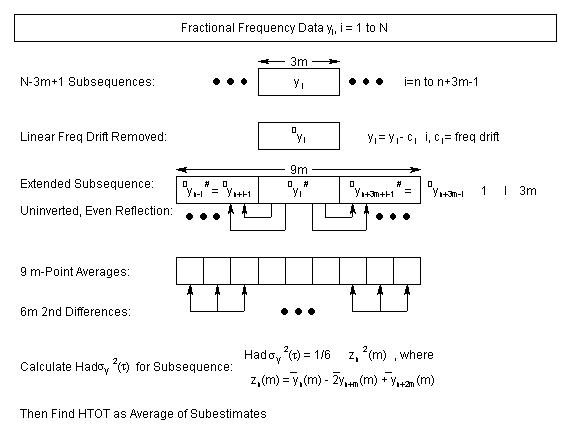Computationally, the HTOT process requires 3 nested loops:

·       An outer summation over the N-3m+1 subsequences.  The 3m-point subsequence is formed, its linear trend is removed, and it is extended at both ends by uninverted, even reflection to 9m points.

·       An inner summation over the 6m unique groups of m-point averages from which all possible fully-overlapping 2nd differences are used to calculate HVAR.

·       A loop within the inner summation to sum the frequency averages for 3 sets of m points.

The final step is to scale the result according to the sampling period, t0, averaging factor, m, and number of points, N.  Overall, this can be expressed as: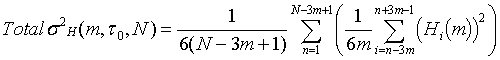where the Hi(m) terms are the zn(m) Hadamard 2nd differences from the triply-extended, drift-removed subsequences.  For best consistency, the overlapping Hadamard variance is used instead of the Hadamard total variance at m=1.  At the largest possible averaging factor, m = N/3, the outer summation consists of only one term, but the inner summation has 6m terms, thus providing a sizable number of estimates for the variance.  The Hadamard total variance is a biased estimator of the Hadamard variance, so a bias correction is required that is dependent on the power law noise type and number of samples.

EXAMPLES

The following plots shown the improvement in the consistency of the overlapping Hadamard deviation results compared with  the normal Hadamard deviation, and the extended averaging factor range provided by the Hadamard total deviation .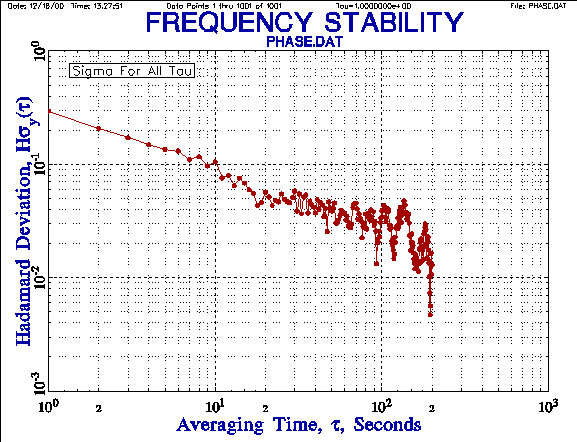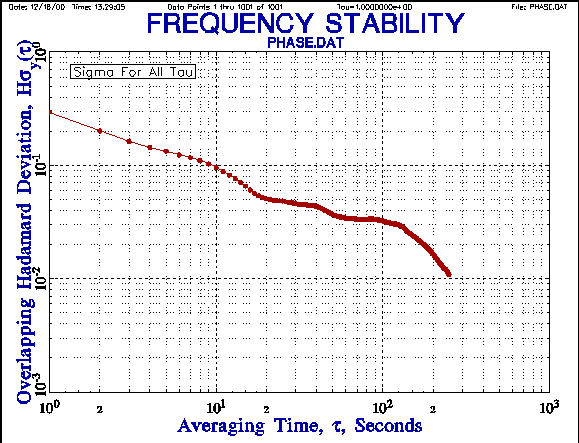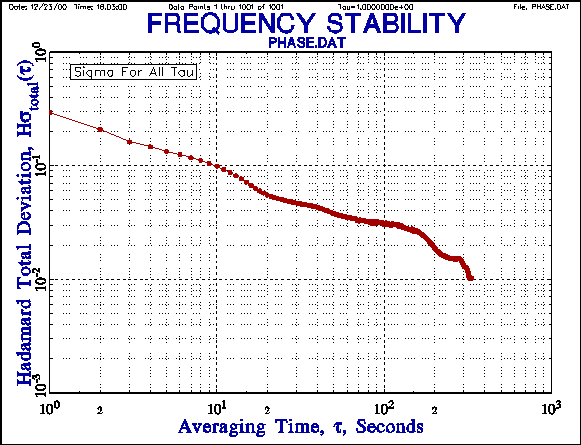A comparison of the overlapping and total Hadamard deviations shows the tighter error bars of the latter, allowing an additional point to be shown at the longest averaging factor.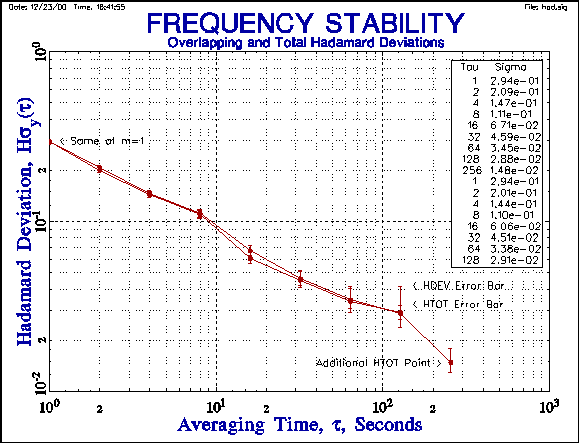TRANSFER FUNCTION

The Hadamard variance sH2(t) may be related to the spectral density of the fractional frequency fluctuations, Sy(f), by its transfer function, |HH(f)|2: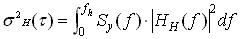where the upper cutoff frequency, fh, is determined by hardware factors.

The transfer function for the 3-sample (N=3) zero dead-time (T=t) binominally-weighted Hadamard variance in the frequency domain is given by [8, 21]: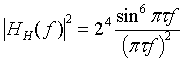which is plotted below: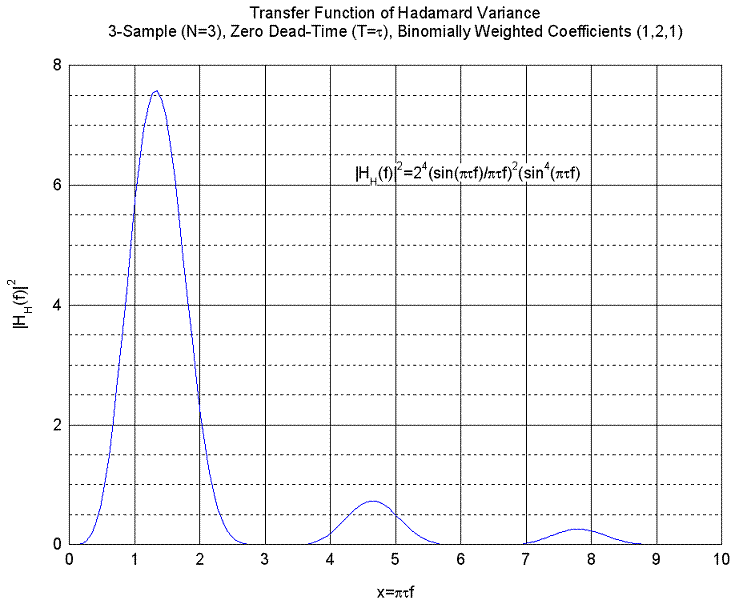For ptf << 1, this function behaves as (ptf)4, showing that the Hadamard variance is convergent for power law noise processes Sya down to as low as a = -4 (Random Run FM).

FREQUENCY DOMAIN ANALYSIS

The Hadamard variance may also be used to perform a frequency domain (spectral) analysis because it has a transfer function that is a close approximation to a narrow rectangle of spectral width of 1/(2·N·t0), where N is the number of samples, and t0 is the measurement time .  This leads to a simple expression for the spectral density of the fractional frequency fluctuations Sy(f) » 0.73 · t0· s2H(t) / N, where f = 1/(2·t0), which can be particularly useful at low Fourier frequencies.

OTHER SIMILAR VARIANCES

The Picinbono variance is a similar 3-sample statistic. It is identical to the Hadamard variance except for a factor of 2/3 [12, 13, 20].  Sigma-z is another statistic that is similar to the Hadamard variance that has been applied to the study of pulsars .

NOISE IDENTIFICATION AND CONFIDENCE LIMITS

It is necessary to identify the dominant power law noise type as the first step in determining the estimated number of chi-squared degrees of freedom for the Hadamard statistics so their confidence limits can be properly set [11, 15].  Because the Hadamard variances can handle the divergent flicker walk FM and random run FM power law noises, techniques for those noise types must be included.  Noise identification is particularly important for applying the bias correction to the Hadamard total variance.

REFERENCES

1.     Jacques Saloman Hadamard (1865-1963), French mathematician.

2.     W.K. Pratt, J. Kane and H.C. Andrews, "Hadamard Transform Image Coding", Proc. IEEE, Vol. 57, No. 1, pp.38-67, January 1969.

3.     R.A. Baugh, "Frequency Modulation Analysis with the Hadamard Variance", Proc. Annu. Symp. on Freq. Contrl., pp. 222-225, June 1971.

4.     K. Wan, E. Visr and J. Roberts, "Extended Variances and Autoregressive Moving Average Algorithm for the Measurement and Synthesis of Oscillator Phase Noise", 43rd Annu. Symp. on Freq. Contrl., pp.331-335, June 1989.

5.     T. Walter, "A Multi-Variance Analysis in the Time Domain", Proc. 24th PTTI Meeting, pp. 413-424, December 1992.

6.     S.T. Hutsell, "Relating the Hamamard Variance to MCS Kalman Filter Clock Estimation", Proc. 27th PTTI Meeting, pp. 291-302, December 1995.

7.     S.T. Hutsell, "Operational Use of the Hamamard Variance in GPS", Proc. 28th PTTI Meeting, pp. 201-213, December 1996.

8.     J. Rutman, "Oscillator Specifications: A Review of Classical and New Ideas", 1977 IEEE International Freq. Contrl. Symp., pp.291-301, June 1977.

9.     This expression for the overlapping Hadamard variance was developed by the author at the suggestion of G. Dieter and S.T. Hutsell.

10.  These plots were produced by the Stable32 program from Hamilton Technical Services.

11.  Private communication, C. Greenhall to W. Riley, 5/7/99.

12.  B. Picinbono, "Processus a Accroissements Stationnaires", Ann. des telecom, Tome 30, No. 7-8, pp. 211-212, July-Aug, 1975.

13.  E. Boileau and B. Picinbono, Statistical Study of Phase Fluctuations and Oscillator Stability, IEEE Trans. Instrum. Meas., IM-25, No. 1, pp. 66-75, March 1976.

14.  D.N. Matsakis and F.J. Josties, "Pulsar-Appropriate Clock Statistics", Proc. 28th PTTI Meeting, pp. 225-236, December 1996.

15.  D. Howe, R. Beard, C. Greenhall, F. Vernotte, and W. Riley, "A Total Estimator of the Hadamard Function Used For GPS Operations", Proc. 32nd PTTI Meeting, Nov. 2000, pp. 255-268.

16.  Chronos Group, Frequency Measurement and Control, Section 3.3.3, Chapman & Hall, London, ISBN 0-412-48270-3, 1994.

17. S. Bregni and L. Jmoda, "Improved Estimation of the Hurst Parameter of Long-Range Dependent Traffic Using the Modified Hadamard Variance", Proceedings of the 2006 IEEE International Conference on Communications (ICC 2006), June 2006 .

18. 2. C.A. Greenhall and W.J. Riley, "Uncertainty of Stability Variances Based on Finite Differences", Proc. 35th PTTI Meeting, December 2003.

19. D.A. Howe, R.L. Beard, C.A. Greenhall, F. Vernotte, W.J. Riley, and T.K. Peppler, "Enhancements to GPS Operations and Clock Evaluations Using a "Total" Hadamard Deviation", IEEE Trans. Ultrason. , Ferroelect., Freq. Contr., Vol. 52, No. 8, August 2005, pp. 1253-1261.

20. J.J Gagnepain, "La Variance de B. Picinbono", Traitement du Signal, Vol. 15, No. 6, Special, 1998, pp. 477-482.

21. J. Rutman, "Characterization of Phase and Frequency Instabilities in Precision Frequency Sources", Proc. IEEE, Vol. 66, No. 9, September1978.

Last Rev. 05/12/07